Courses

# Test: Gaseous State - 5

## 30 Questions MCQ Test Physical Chemistry | Test: Gaseous State - 5

Description
This mock test of Test: Gaseous State - 5 for Chemistry helps you for every Chemistry entrance exam. This contains 30 Multiple Choice Questions for Chemistry Test: Gaseous State - 5 (mcq) to study with solutions a complete question bank. The solved questions answers in this Test: Gaseous State - 5 quiz give you a good mix of easy questions and tough questions. Chemistry students definitely take this Test: Gaseous State - 5 exercise for a better result in the exam. You can find other Test: Gaseous State - 5 extra questions, long questions & short questions for Chemistry on EduRev as well by searching above.
QUESTION: 1

Solution:
QUESTION: 2

### Volume of the air that will be expelled from a vessel of 300 cm3 when it is heated from 27°C to 37°C at the same pressure will be:

Solution:

V1/T1 = V2/T2

V2 = V1T2/T1 = (300 x 310)/300 = 310 cm3

Volume of air expelled = 310 cm3 - 300 cm3 = 10 cm3

QUESTION: 3

### 2.8 g of a gas at 1 atm and 273 K occupies a volume of 2.24 litres, the gas can not be:

Solution:
QUESTION: 4

Calculate the volume of O2 at 1 at m and 273 K required for the complete combustion of 2.64 L of acetylene (C2H2) at 1 atm and 273 K. 2C2H2(g) + 5O2(g) → 4CO2(g) + 2H2O(l)

Solution: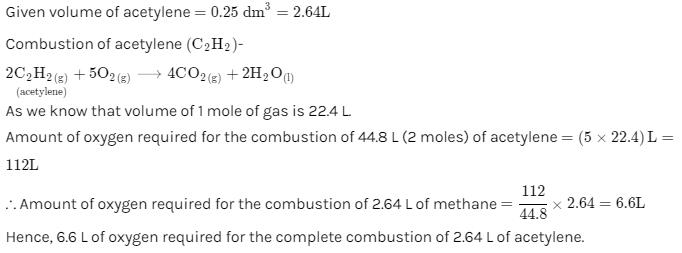QUESTION: 5

A certain hydrate has the formula MgSO4.xH2O. a quantity of 54.2 g of the compound is heated in an over to drive off the water. If the steam generated exerts a pressure of 24.8 atm in a 2.0 L container at 120°C, calculate x:

Solution:
QUESTION: 6

A high altitude balloon contains 6.81 g of helium in 1.16 × 104 L at –23°C. Assuming ideal gas behaviour, how many grams of helium would have to be added to increase the pressure to 4.0 × 10–3 atm:

Solution:

P1 = 6.81/4 × (0.0821 × 250)/(1.1 × 104)
= 3.012 × 10-3
Pf = 4 × 10-3
△P↑⏐= 1 × 10-3
nHe - added = (10-3 × 1.16 × 104)/(0.0821 × 250)
wt = nHe × 4 = 2.24gm.

QUESTION: 7

Starting out on a trip into the mountains, you inflate the tires on your automobile to a recommended pressure of 3.21 × 105 Pa on a day when the temperature is –5°C. You drive to the beach, where the temperature is 28.0°C. Assume that the volume of the tire has increased by 3%. What is the final pressure in the tyres:

Solution: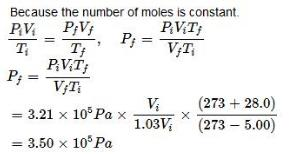QUESTION: 8

Two glass bulbs A and B at same temperature are connected by a very small tube having a stop-cork. Bulb A has a volume of 100 cm3 and contained the gas while bulb B was empty. On opening the stop-cork, the pressure fell down to 20%. The volume of the bulb B is:

Solution:

We know that, at constant temperature, PV = constant

So, P1V1 = P2V2

Or, P1/P2 = V2/ V1

Since, P2= (P1 - 20% P1) = 4/5P1,

Let, volume of bulb B is = V, i.e. V1= V cm3,

Now, after opening the cork, the volume of bulb A is also added and new volume becomes,  V2= (V + 100) Cm3

Hence, new volume of bulb B, V2 =   (P1/P2) x V1 = [P1/(4/5P1)]x V1

Or, putting the values we get,

(V + 100) = 5P1/4P1 x V = 5V/4

So, 5V = 4(V+100) = 4V + 400

Hence, original volume of bulb B, V = 400 cm3

QUESTION: 9

Two closed vessel A and B of equal volume of 8.21 L are connected by a narrow tube of negligible volume with open valve. The left hand side container is found to contain 3 mole CO2 and 2 mole of He at 400 K, what is the partial pressure of He in vessel B at 500 K:

Solution:

partial pressure of He in vessel B,

PV = nRT

PHe = nRT/V

(2×8.314×400​)/8.21

= 8atm

QUESTION: 10

56 g of nitrogen and 96 g of oxygen are mixed isothermally and at a total pressure of 10 at m. The partial pressures of oxygen and nitrogen (in atm) are respectively:

Solution:

Raoult's Law states that the partial vapour pressure of a component in a mixture is equal to the vapour pressure of the pure component at that temperature multiplied by its mole fraction in the mixture.

In a mixture of liquids of A and B,

pA​=xA​×p

pB​=xB​×p

moles of nitrogen gas is nN2​​=56/28=2 mol

moles of oxygen gas is nO2​​=96/32=3 mol

Mole fraction of nitrogen is xN2​​=2/(2+3)=0.4

Mole fraction of oxygen will be xO2​​=1−0.4=0.6

Partial pressure of oxygen is pO2​​=0.6×10=6 atm

Partial pressure of Nitrogen is pN2​​=0.4×10=4 atm

QUESTION: 11

Oxygen gas generated by the decomposition of potassium chlorate is collected over water. The volume of oxygen collected at 24°C and atmospheric pressure of 760 mmHg is 128 mL. Calculate the mass of oxygen gas obtained. The pressure of the water vapour at 24°C is 22.4 mmHg:

Solution: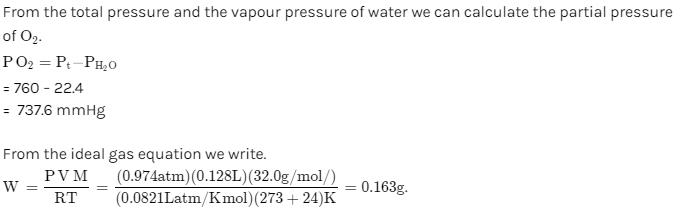QUESTION: 12

Consider three one-litre flasks labeled A, B and C filled with the gases NO, NO2 and N2O, respectively, each at 1 atm and 273 K. In which flask do the molecules have the highest average kinetic energy:

Solution: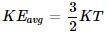QUESTION: 13

Two flasks A and B have equal volumes. A is maintained at 300 K and B at 600 K, while A contains H2 gas, B has an equal mass of CO2 gas. Find the ratio of total K.E. of gases in flask A to that of B:

Solution: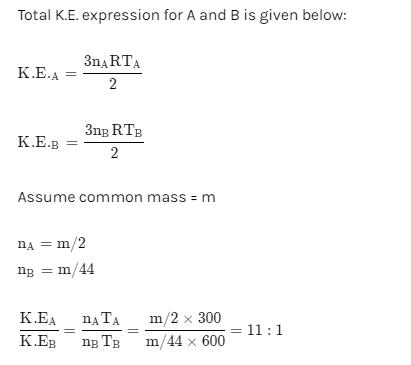QUESTION: 14

A mixture of Ne and Ar at 250 K has a total K.E. = 3 kJ in a closed vessel, the total mass of Ne and Ar is 30 g. Find mass % of Ne in gaseous mixture at 250 K

Solution:

K = 3/2nTRT
⇒ nT = (3000 × 2)/(3 × 250 × 8.314)
3 × 103 = 3/2nT × 8.314 × 250
x/20 + (30 − x)/40

= 2/8.314
% Ne = 28.3

QUESTION: 15

The most probable speed of 8 g of H2 200 ms–1 average kinetic energy (neglect rotational and vibrational energy) of H2 gas is:

Solution:
QUESTION: 16

The root mean square speed of 8 g of He is 300 ms–1. Total kinetic energy of He gas is:

Solution:
QUESTION: 17

The ratio among most probable velocity, mean velocity and root mean square velocity is given by:

Solution:
QUESTION: 18

Calculate relative rate of effusion of O2 to CH4 through a container containing O2 and CH4 in 3:2 mass ratio:

Solution:

rO2/rCH4 = nO2/nCH4.(√MCH4/MO2
= 3/2 × 16/32 × (√16/32)
= 3/(4√2)

QUESTION: 19

Calculate γ (ratio of Cp and Cv) for triatomic linear gas at high temperature. Assume that the contribution of vibrational degree of freedom is 75%:

Solution:
QUESTION: 20

If one mole each of a monoatomic and diatomic gases are mixed at low temperature then Cp/Cv ratio for the mixture is:

Solution:
QUESTION: 21

At low pressures, van der Waal’s equation is written as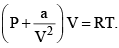The compressibility factor is then equal to:

Solution:
QUESTION: 22

The temperature at which the second virial coefficient of real gas is zero is called:

Solution:
QUESTION: 23

The excluded volume of a gas will be larger, if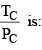Solution:
QUESTION: 24

A mixture of NH3(g) and N2H4(g) is placed in a sealed container at 300 K. The total pressure is 0.5 atm. The container is heated to 1200 K at which time both substances decompose completely according to the equations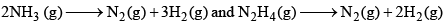After decomposition is complete, the total pressure at 1200 K is found to be 4.5 atm. Find the mole % of N2H4 in the original mixture:

Solution:
QUESTION: 25

A given volume of ozonised oxygen (containing 60% oxygen by volume) required 220 sec to effuse which an equal volume of oxygen took 200 sec only under the conditions. If density of O2 is 1.6 g/L then find density of O3:

Solution:
QUESTION: 26

The van der Waal’s constant ‘b’ of a gas is 4π × 10–4 L/mol. How near can the centres of the two molecules approach each other? [Use: NA = 6 × 1023]

Solution:
QUESTION: 27

At a constant pressure, what should be the percentage increase in the temperature in Kelvin for a 10% increase in volume:

Solution:
QUESTION: 28

For a real gas (mol. mass = 60) if density at critical point is 0.80 g/cm3 and its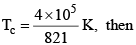van der Waal’s constant a (in at m L2 mol–2) is:

Solution:
QUESTION: 29

A mixture of nitrogen and water vapours is admitted to a flask at 760 torr which contains a sufficient solid drying agent after long time the pressure reached a steady value of 722 torr. If the experiment is done at 27°C and drying agent increases in weight by 0.9 gm, what is the volume of the flask? Neglect any possible vapour of drying agent and volume occupied by drying agent:

Solution:
QUESTION: 30

Vander Waal’s gas equation can be reduced to virial equation and virial equation (in terms of volume)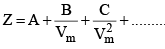Where A = first virial coefficient, B = second virial coefficient, C = third virial coefficient. The third virial coefficient of Hg(g) is 625 (cm2/mol)2. What volume is available for movement of 10 moles He(g) atoms present in 50 L vessel:

Solution: